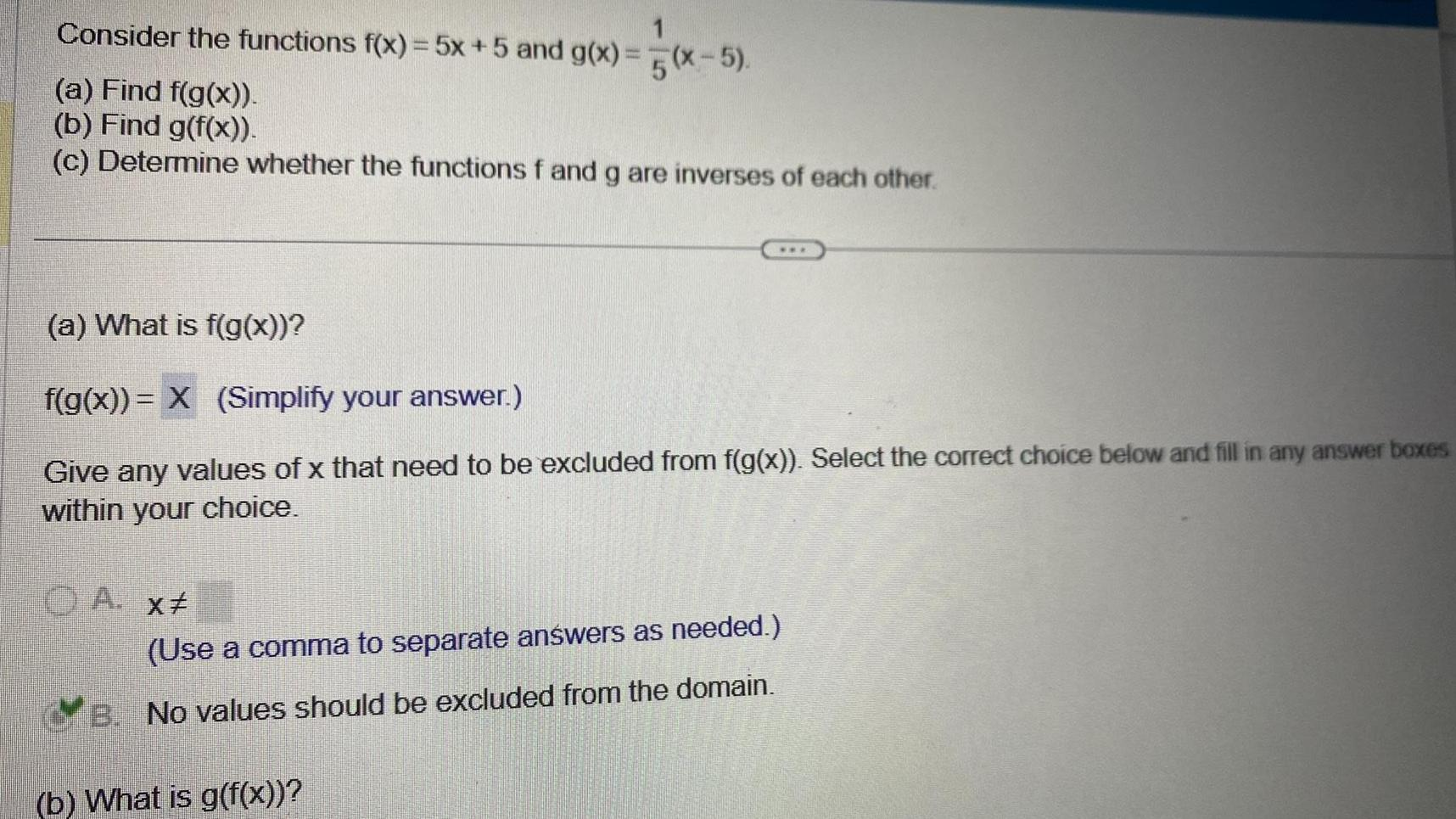Question:

# 1 Consider the functions f x 5x 5 and g x 5 x 5 a Find f g x

Last updated: 11/20/20231 Consider the functions f x 5x 5 and g x 5 x 5 a Find f g x b Find g f x c Determine whether the functions f and g are inverses of each other a What is f g x f g x X Simplify your answer Give any values of x that need to be excluded from f g x Select the correct choice below and fill in any answer boxes within your choice A x Use a comma to separate answers as needed B No values should be excluded from the domain b What is g f x